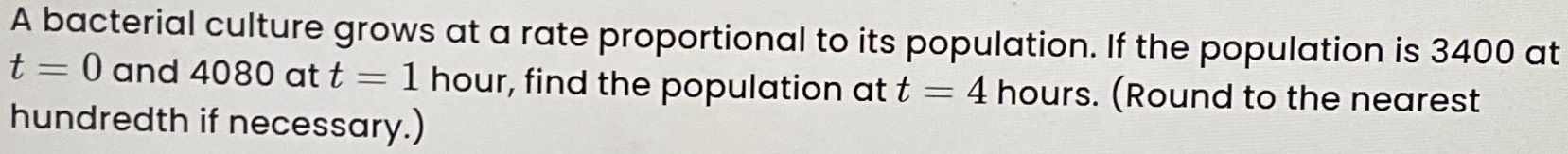### ¿Todavía tienes preguntas de matemáticas?

Pregunte a nuestros tutores expertos
Algebra
PreguntaA bacterial culture grows at a rate proportional to its population. If the population is $$3400$$ at

$$t = 0$$ and $$4080$$ at $$t = 1$$ hour, find the population at $$t = 4$$ hours. (Round to the nearest hundredth if necessary.)

$$y= 680x+ 3400$$
$$x= 1,y= 6120$$